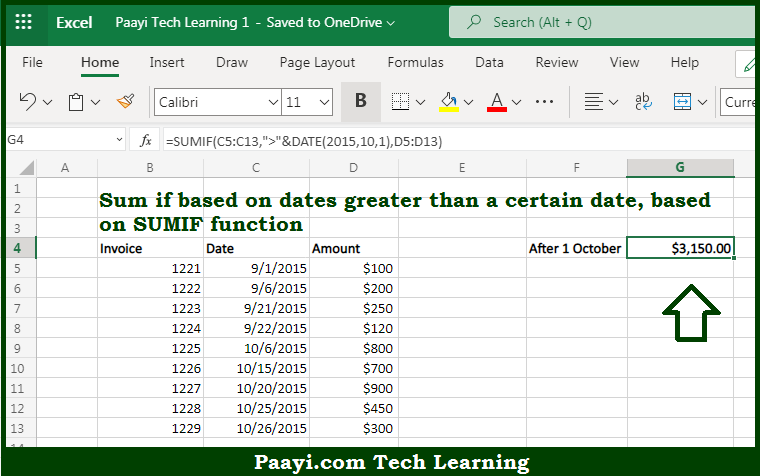# Learn How to SUM If Date is Greater Than in Microsoft Excel

Written by | 0 Comments | 493 Views

In this article, you will learn how to SUM various things in Microsoft Excel using a single/combination(s) of functions. You will also know how to SUM If Date is Greater Than and see the generic formula.

SUM If Date is Greater Than in Microsoft Excel

The main purpose of this formula is to sum if based on dates greater than a certain date. Here we will learn how to sum the if the date is greater than some other date in the workbook in Microsoft Excel. That implies, with the help of a formula based on the SUMIF function you can able to sum if based on dates greater than a certain date. So, with the help of this formula, you can able to sum the if the date is greater than some other date in the workbook in Microsoft Excel.

General Formula to SUM If Date is Greater Than

=SUMIF(range,">"&DATE(year,month,day),sum_range)

The Explanation for the SUM If Date is Greater ThanSo we know that with the help of the given formula above you can able to sum if based on dates greater than a certain date. Here we will learn how to sum the if the date is greater than some other date in the workbook in Microsoft Excel. As we know that the UMIF function supports  logical operators efficiently, which are -  "=",">",">=", etc. So you can able to use these operators as per your criteria. Moreover, the DATE function is a safe way to create dates for function criteria because it eliminates problems associated with regional date settings. It should be noted that to enclose the greater-than the operator in double-quotes and join it to the date with an ampersand (&). So, with the help of this formula, you can able to sum the if the date is greater than some other date in the workbook in Microsoft Excel.